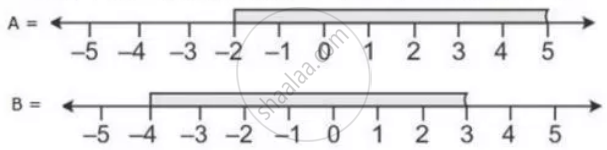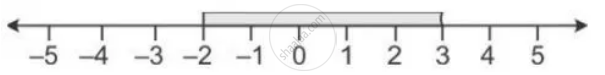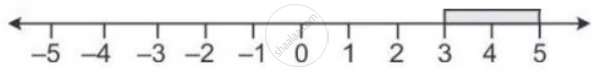Share

The Diagram Represents Two Inequations a and B on Real Number Lines: 1) Write Down a and B in Set Builder Notation/ 2) Represent a ∪ B and A ∩ B' on Two Different Number Lines - ICSE Class 10 - Mathematics

ConceptRepresentation of Solution on the Number Line

Question

The diagram represents two inequations A and B on real number lines:1) Write down A and B in set builder notation/

2) Represent A ∪ B and A ∩ B' on two different number lines

Solution

1) A = {x ∈ R: -2 ≤ x < 5}

B = {x ∈ R: -4 ≤ x < 3}

2) A ∩ B = {x ∈ R: -2 ≤ x < 5}

It can be represented on number line as:B’ = {x ∈ R: 3 < x ≤ -4}

A ∩ B’ = {x ∈ R: 3 ≤ x < 5}

It can be represented on number line as:Is there an error in this question or solution?

APPEARS IN

Solution The Diagram Represents Two Inequations a and B on Real Number Lines: 1) Write Down a and B in Set Builder Notation/ 2) Represent a ∪ B and A ∩ B' on Two Different Number Lines Concept: Representation of Solution on the Number Line.
S